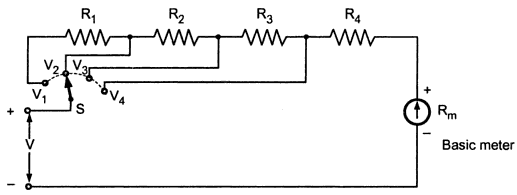### Multirange Voltmeter

The range of the basic d.c. voltmeter can be extended by using number of multipliers and a selector switch. Such a meter is called multirange voltmeter and is shown in the Fig. 1.Fig.1 multirange voltmeter

The R1, R2, R3 and R4 are the four series multipliers. When connected in series with the meter, they can give four different voltage ranges as V1, V2, Vand V4. The selector switch S is multiposition switch by which the required multiplier can be selected in the circuit.
The mathematical analysis of basic d.c. voltmeter is equally applicable for such multirange voltmeter. Thus,

1.1 Practical Multirange Voltmeter
More practical arrangement of multiplier resistances is shown in the Fig.2.Fig. 2

In this arrangement, the multipliers are connected in series string. The connections are brought out from the junctions of the resistances. The selector switch is used to select the required voltage range.
When the switch S is at position V1,  R+ R+ R+ R4 acts as a multiplier resistance. While when the switch S is at position Vthen the resistance Ronly acts as multiplier resistance. The V4 is the lowest voltage range while Vis the maximum voltage range.
The multiplier resistances can be calculated as :
In position V4, the multiplier is Ronly. The total resistance of the circuit is say R.

In position V3, the multiplier is RR4.

In position V2, the multiplier is RRR4.

In position V1, the multiplier is RRRR4.

Using equations (1), (2), (3) and (4) multipliers can be designed. The advantage of this arrangement is that the multiplier except Rhave standard resistance values and can be obtained commercially on precision tolerances. The first resistance i.e. Ronly is the resistance having special value and must be manufactured specially to meet the circuit requirements.